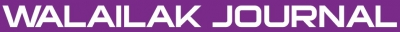Accuracy of VIM, HPM and ADM in Solving Nonlinear Equations for the Steady Three-Dimensional Flow of a Walter’s B Fluid in Vertical Channel

Abstract

In this paper, the steady three-dimensional flow of a Walter's B fluid in a vertical channel is investigated. It is assumed that the fluid is injected into the passage through one side of the channel. The combined effects of viscoelasticity and inertia are considered. By using the appropriate similarity transformations for the velocity components and temperature, the basic equations governing flow and heat transfer are reduced to a set of ordinary differential equations. These equations are solved approximately, subject to the relevant boundary conditions, with a numerical technique. In the present study, three powerful analytical methods of Variational iteration method (VIM), Homotopy perturbation method (HPM) and Adomian decomposition method (ADM) are introduced to overcome this shortcoming. Then, VIM, HPM and ADM are used to solve nonlinear equations in fluids. These methods are useful and practical for solving the nonlinear equation in fluids. Comparison of the results obtained by all three methods and exact solutions reveals that all three methods are tremendously effective.

doi:10.14456/WJST.2014.12

Keywords

Flow of a Walter’s B fluid, Adomian decomposition method (ADM), Variational iteration method (VIM), Homotopy perturbation method (HPM)

PDF

References

MA Abdou and AA Soliman. Variational iteration method for solving Burger’s and coupled Burger’s equations. J. Comput. Appl. Math. 2005; 181, 245-51.

EM Abulwafa, MA Abdou and AA Mahmoud. The solution of nonlinear coagulation problem with mass loss. Chaos Soliton. Fract. 2006; 29, 313-30.

A Aziz and TY Na. Perturbation Method in Heat Transfer. Hemisphere Publishing Corporation, Washington DC, 1984.

N Nadim and G Domairry. Analytical study of natural convection in high Prandtl number. Energ. Convers. Manag. 2009; 50, 1056-61.

Z Ziabakhsh and G Domairry. Analytic solution of natural convection flow of a non-Newtonian fluid between two vertical flat plates using homotopy analysis method. Comm. Nonlinear Sci. Numer. Simulat. 2009; 14, 1868-80.

DD Ganji and A Rajabi. Assessment of homotopy perturbation and perturbation methods in heat radiation equations. Inter. Comm. Heat Mass Tran. 2006; 33, 391-400.

Y Cherruault. Convergence of Adomian’s method. Kybernetes 1989; 18, 31-8.

Y Cherruault and G Adomian. Decomposition methods. a new proof of convergence. Math. Comput. Model. 1993; 18, 103-6.

K Abbaoui and Y Cherruault. Convergence of Adomian’s method applied to differential equations. Comput. Math. Appl. 1994; 28, 103-9.

K Abbaoui and Y Cherruault. New ideas for proving convergence of decomposition methods. Comput. Math. Appl. 1995; 29, 103-8.

JH He. Homotopy perturbation technique. Comput. Meth. Appl. Mech. Eng. 1999; 178, 257-62.

JH He. Variational iteration method for autonomous ordinary differential systems. Appl. Math. Comput. 2000; 114, 115-23.

JH He. A coupling method of a homotopy technique and a perturbation technique for non-linear Problems. Int. J. Nonlinear Mech. 2000; 35, 37-43.

JH He. Homotopy perturbation method, a new nonlinear analytical technique. Appl. Math. Comput. 2003; 135, 73-9.

Z Ziabakhsh and G Domairry. Solution of the laminar viscous flow in a semi-porous channel in the presence of a uniform magnetic field by using the homotopy analysis method Original Research Article. Comm. Nonlinear Sci. Numer. Simulat. 2010; 14, 37-43.

JH He. Application of homotopy perturbation method to nonlinear wave equations. Chaos Soliton. Fract. 2005; 26, 695-700.

JH He. Homotopy perturbation method for bifurcation of nonlinear problems. Int. J. Nonlinear Sci. Numer. Simulat. 2005; 6, 207-8.

JH He. Some asymptotic methods for strongly nonlinear equations. Int. J. Mod. Phys. 2006; 20, 1141-99.

AA Joneidi, G Domairry and M Babaelahi. Three analytical methods applied to Jeffery-Hamel flow. Comm. Nonlinear Sci. Numer. Simulat. 2010; 15, 3423-34.

JH He and XH Wu. Construction of solitary solution and compacton-like solution by variational iteration method. Chaos Soliton. Fract. 2006; 29, 108-13.

S Momani and S Abuasad. Application of He’s variational iteration method to Helmholtz equation. Chaos Soliton. Fract. 2006; 27, 1119-23.

ZM Odibat and S Momeni. Application of variational iteration method to nonlinear differential equations of fractional order. Int. J. Nonlinear Sci. Numer. Simulat. 2006; 7, 27-34.

S Pamuk. Solution of the porous media equation by Adomian’s decomposition method. Phys. Lett. 2005; 344, 184-8.

AD Polyanin and V F Zaitsev. Handbook of Nonlinear Partial Differential Equations. Chapman & Hall, CRC Press, Boca Raton, 2004.

AM Siddiqui, M Ahmed and QK Ghori. Couette and Poiseuille ﬂows for non-Newtonian ﬂuids. Int. J. Nonlinear Sci. Numer. Simulat. 2006; 7, 15-26.

AM Siddiqui, R Mahmood and QK Ghori. Homotopy perturbation method for thin film flow of a fourth grade fluid down a vertical cylinder. Phys. Lett. A 2006; 352, 404-10.

MM Rashidi, G Domairry and S Dinarvand. Approximate solutions for the Burger and regularized long wave equations by means of the homotopy analysis method. Comm. Nonlinear Sci. Numer. Simulat. 2009; 14, 708-17.

AA Soliman. A numerical simulation and explicit solutions of KdV-Burgers’ and Lax’s seventh-order KdV equations. Chaos Soliton. Fract. 2006; 29, 294-302.

Refbacks

• There are currently no refbacks.Online ISSN: 2228-835X

http://wjst.wu.ac.th

Last updated: 17 May 2019